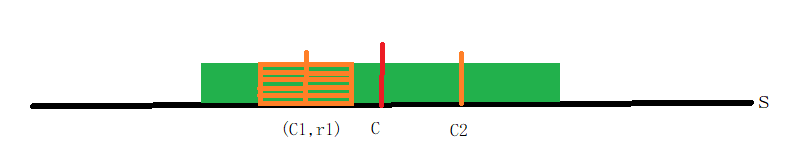# 最长回文子串Manacher算法

while (s[c+r] == s[c-r]) {
r = r + 1;
}// maxCover:最右边界 maxC:最右边界对应的中心 r[]:每个点作为中心的半径
curR = 1;
if (C2 < maxCover) {
C1 = maxC - (C2 - maxC);
r1 = r[C1];
curR = min(r1, maxCover - C2);
}
while (s[C2 - curR] == s[C2 + curR]) {
curR = curR + 1;
}
curR = curR - 1;

// 维护 maxCover 等

©️2019 CSDN 皮肤主题: 编程工作室 设计师: CSDN官方博客